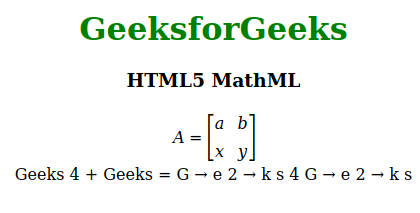GeeksforGeeks App
Open AppBrowser
Continue

# HTML5 | MathML Introduction

The MathML comes in HTML5 the current MathML version is 3 it was introduced in the year of 2015. MathML stands for Mathematics Markup Language. It is used to represent mathematical equations or expressions in web browsers like other HTML elements. The 1st version of MathML was released in the year of 1998 and after that in the 2nd version was released.

Basically, MathML is a complex mathematical formula or equation visual representation made easy. The MathML is supported in the HTML5, all the MathML tag must be used inside the <math> and </math> tags. The MathML is used to describe mathematics as a basis for the machine to machine communication, it is intended handling by specialized authoring tools such as equation editors and it is meaningful to other applications also.

MathML is not-capable:

• The MathML is not a calculator to calculate or solve complex equation it is just a way to show the equation.
• It is not a programming language it is a Mathematics Markup Language.

MathML is capable:

• The MathML can be used to represent the Matrix form.
• The MathML can be used to represent the Partial Differential Equation.
• The MathML can be used to represent the Chemical Reaction Equation.

Below example gives you a brief idea about MathML

Example:

## html

 ```<``html``>` `<``head``>``    ``<``title``>HTML5 MathML``` `<``body` `style="text-align:center;">` `    ``<``h1` `style="color:green">``        ``GeeksforGeeks``    ``` `    ``<``h3``>HTML5 MathML` `    ````    ``<``math``>``        ` `        ````        ``<``mrow``>``            ``<``mi``>A``            ``<``mo``>=` `            ``<``mfenced` `open="[" close="]">` `                ``<``mtable``>``                    ``<``mtr``>``                        ``<``mtd``>``                            ``<``mi``>a``                        ````                        ``<``mtd``>``                            ``<``mi``>b``                        ````                    ``` `                    ``<``mtr``>``                        ``<``mtd``>``                            ``<``mi``>x``                        ````                        ``<``mtd``>``                            ``<``mi``>y``                        ````                    ``` `                ``` `            ````        ````      ` `        ````        ``<``br``><``br``>``        ``<``msub``>``            ``<``mi``>Geeks``            ``<``mn``>4``        ````        ``<``mo``>+``        ``<``mn``>Geeks``        ``<``mo``>=``        ``<``msub``>``            ``<``mi``>G``        ````        ``<``mo``>→``        ``<``msub``>``            ``<``mi``>e``            ``<``mn``>2``        ````        ``<``mo``>→``        ``<``mi``>k``        ``<``mi``>s``        ``<``mn``>4``        ````        ``<``msub``>``            ``<``mi``>G``        ````        ``<``mo``>→``        ``<``msub``>``            ``<``mi``>e``            ``<``mn``>2``        ````        ``<``mo``>→``        ``<``mi``>k``        ``<``mi``>s``    ````` ``

Output:Note: There are almost 30+ MathML tags available. All the tags are listed below in table format:

Supported Browsers: The browsers supported by HTML5 MathML tags are listed below:

• Firefox

My Personal Notes arrow_drop_up
Related Tutorials